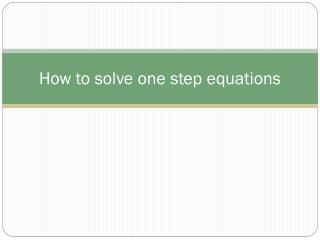# How to solve one step equations - PowerPoint PPT PresentationDownload PresentationHow to solve one step equations

How to solve one step equationsDownload Presentation## How to solve one step equations

- - - - - - - - - - - - - - - - - - - - - - - - - - - E N D - - - - - - - - - - - - - - - - - - - - - - - - - - -
##### Presentation Transcript

1. How to solve one step equations

2. What’s up with the variable • What ever is happening with the variable, you must do the opposite. • For example if the variable is being added to something you must subtract to solve

3. You try • What is happening and what will you use to solve it? • X + 10 • (5)(Y)

4. Take action • Once you know which operation to use use to solve • Then perform that operation to both sides of the equation • Remember we have to stay balanced in math

5. You try • (7)(A)=49 • 45 + M=60